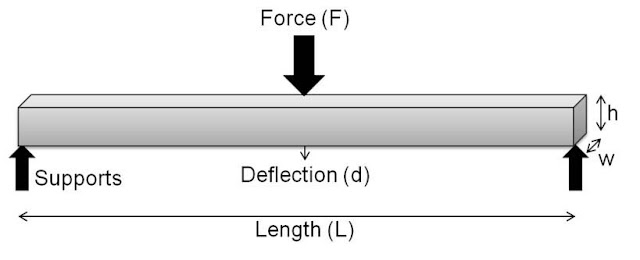# TOS ~ Moment Distribution Method - Ishwaranand

## TOSMoment Distribution Method

### Introduction of Moment Distribution Method

• Suggested by Prof. Hardy Cross in the early 1930s.
• The method is widely used for the analysis of indeterminate structures.
• Ideally suited for a fairly higher degree of indeterminate structuresImage Source ~ Crafted With ©Ishwaranand - 2020 ~ Image by ©Ishwaranand

### Indeterminate structures of Moment Distribution Method

• These are the structures which cannot be fully analysed by the condition of equilibrium.
• The equations of statical equilibrium which are based on Sir Isaac Newton’s law governing the motion of bodies 1687 which are
1. The summation of all forces in any axis is zero.
2. The summation of all moment in any axis is zero.
If summation is taken about x, y and z axes, symbolically this becomes.
• ∑Fx = 0;    ∑Fy = 0;  and  ∑Fz = 0;
• ∑Mx = 0;   ∑My = 0; and  ∑Mz = 0;
For Planar structures and forces acting in the same plane, the equation reduces to
• ∑Fx = 0, ∑Fy =  0, and  ∑Mz = 0,

### Types of Supports in Moment Distribution Method

#### Calculation of Indeterminacy by Formulae

• Degree of Indeterminacy of Frame: i=(3m +r) – (3j +c)
• Or statically determinate if 3j = 3m +r
• Degree of Indeterminacy of Plane Truss: i=(2j -3) – m
• Or statically determinate if m=2j -3Image Source ~ Crafted With ©Ishwaranand - 2020 ~ Image by ©Ishwaranand

### Terminology on Moment Distribution Method

#### Stiffness

• The moment required to rotate an end by the unit angle 1 radian, when rotation is permitted at that end, is called stiffness of the beam. Thus in the beam above, if θA is the rotation at end A,
• Stiffness of the beam AB = k (M/ θA)
OR

### Distribution factor of Moment Distribution Method

• If a moment is connected to a rigid joint where a number of members are meeting, the connected moment is shared with the branches meeting about that joint.
• The ratio of the moment shared by a member to the applied moment at the joint is called the distribution factor of that member.
• Thus, if MoA is the moment shared by member OA when moment M is applied at joint O, then the distribution factor for member OA is dOA = MOA/MImage Source ~ Crafted With ©Ishwaranand - 2020 ~ Image by ©Ishwaranand

### Application of Moment Distribution Method to Continuous Beams with Fixed ends

• Assuming all ends are fixed, find the fixed end moment s developed.
• Calculate distribution factors for all members meeting at a joint.
• Balance a joint by distributing balance moment to various members meeting at the joint proportional to their distribution factors. Do a similar exercise for all joints.
• Carryover half the distributed moment to the far ends of the members. This upsets the balances of the joints.
• Repeat steps 3 and 4 till distributed moment are negligible.
• Sum up all the moments at a particular end of the member to get a final moment.